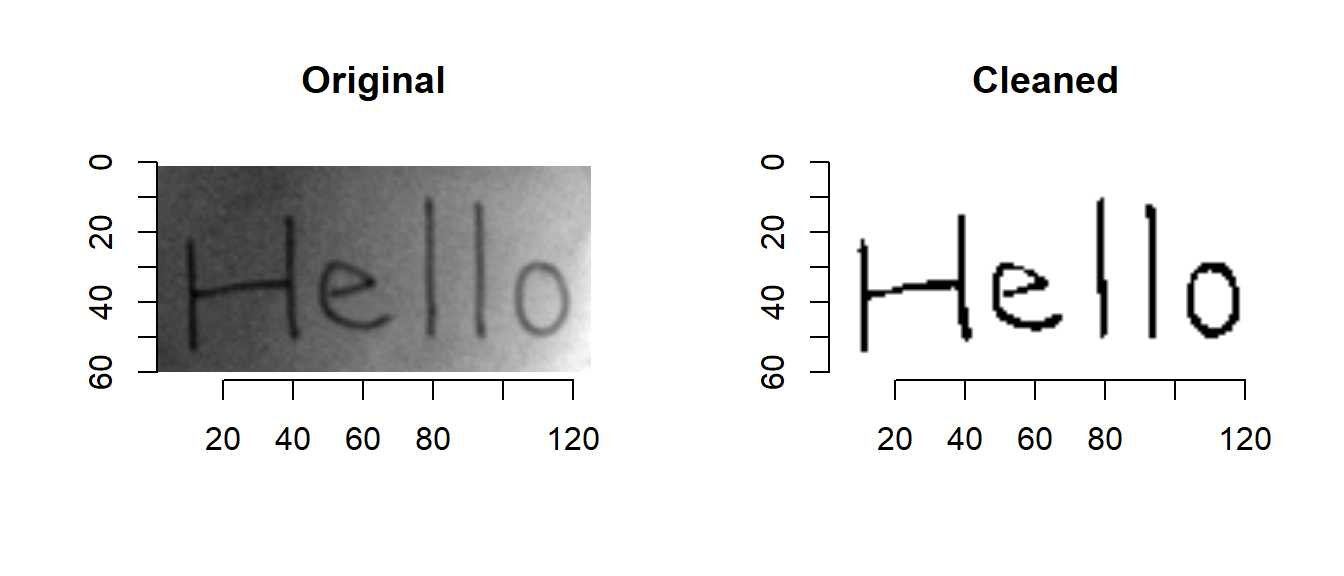Optical Character Recgnition with imagerExtra

We can do optical character recgnition by using the R package tesseract.

ocr function of tesseract works best for images with high contrast, little noise and horizontal text.

Then, how should we extract text from a degraded image?

A way is that we enhance contrast of image and denoise image with imagerExtara before using ocr function.

It’s convenient we have a function that is shortcut to ocr function if we adopt the way.

That’s why I implemented the functions (OCR and OCR_data) that are shortcuts to ocr function and ocr_data function of tesseract.

You can use them as show below.

library(devtools)
install_github("ShotaOchi/imagerExtra")
library(imagerExtra)
layout(matrix(1:2, 1, 2, byrow=TRUE))
plot(papers, main = "Original")
hello <- DenoiseDCT(papers, 0.01) %>% ThresholdAdaptive(., 0.1, range = c(0,1)) %>% plot(main = "Cleaned")OCR(hello) %>% cat
Hello
OCR_data(hello)
# A tibble: 1 x 3
word  confidence bbox
<chr>      <dbl> <chr>
1 Hello       69.1 8,9,118,54